##Circle Bundles

The first example of a [circle bundle] is the set of all circles passing through two points A and B (the green circles). All pairs of circles of this bundle share the same [radical axis], which is the line [AB]. Therefore some times the name (coaxal system of circles). There is a smallest circle in this bundle, having as diameter the segment [AB]. There is no biggest circle in this bundle. With growing radius the circle tends to the common radical axis, line [AB].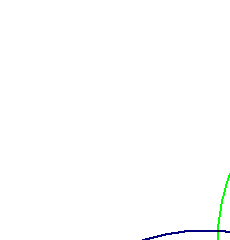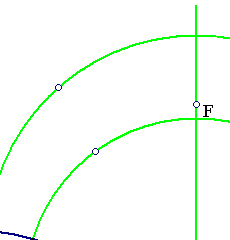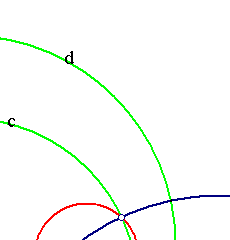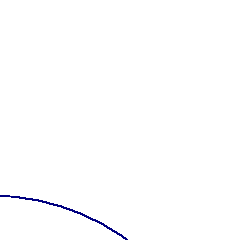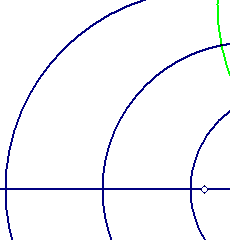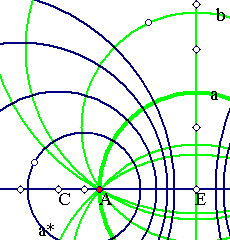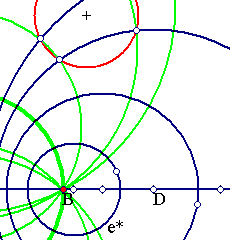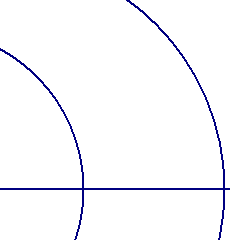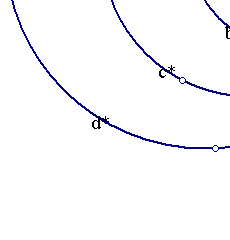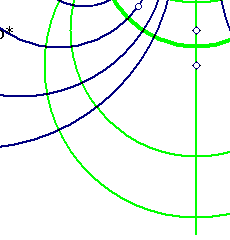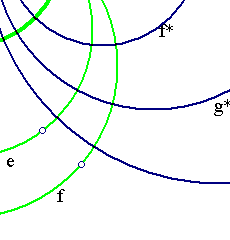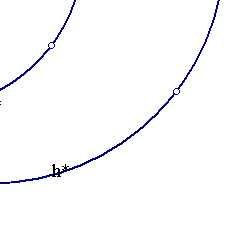Closely related to the first bundle (one says [of intersecting type]) is the other bundle (the blue one). This second bundle is the set of all possible circles orthogonal to two members of the first bundle. One shows easily that then each member of the second bundle is orthogonal to every circle of the first bundle. The pairs of circles of the second bundle share the same radical axis which is the line of the centers of the circles of the first bundle. There is no minimal circle in this bundle, but there are arbitrary small circles, tending to points A and B. These are called [limiting points] of the bundle. No pair of circles of this bundle intersect (therefore one speaks of non-intersecting type). Again there is no maximal circle in this bundle. With growing radius the circles of the bundle tend to the common radical axis, which is the medial line of segment AB. See the file CircleBundleTangent.html for a third kind of a circle bundle. Exercise: state and prove the result indicated by the red circle in the above figure.

By letting B go to infinity we obtain the following case of orthogonal bundles, in which the green bundle consists of lines, considered as limit cases of circles through A. The bundle above transforms to the bundle below through the inversion whose center is at B and the radius is BA.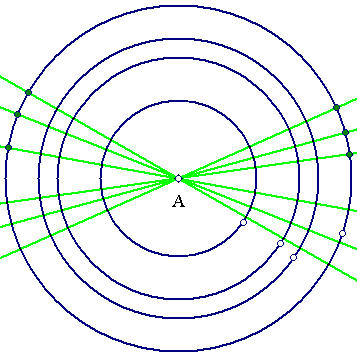More general Moebius transformations respect the set of {circles + lines} and are conformal i.e. preserve angles, hence map orthogonal bundles of circles to orthogonal bundles of circles. Here we can allow generalized bundles of circles consisting also from lines, passing through a fixed point (as above) or being parallel.

### See Also

CircleBundleTangent.html
InvertToCanonical.html

 Produced with EucliDraw© http://users.math.uoc.gr/~pamfilos/